A voltage to frequency converter can realize an opto-isolated analog input for Arduino or other microcontroller systems.

IntermediateFull instructions provided5 hours8,578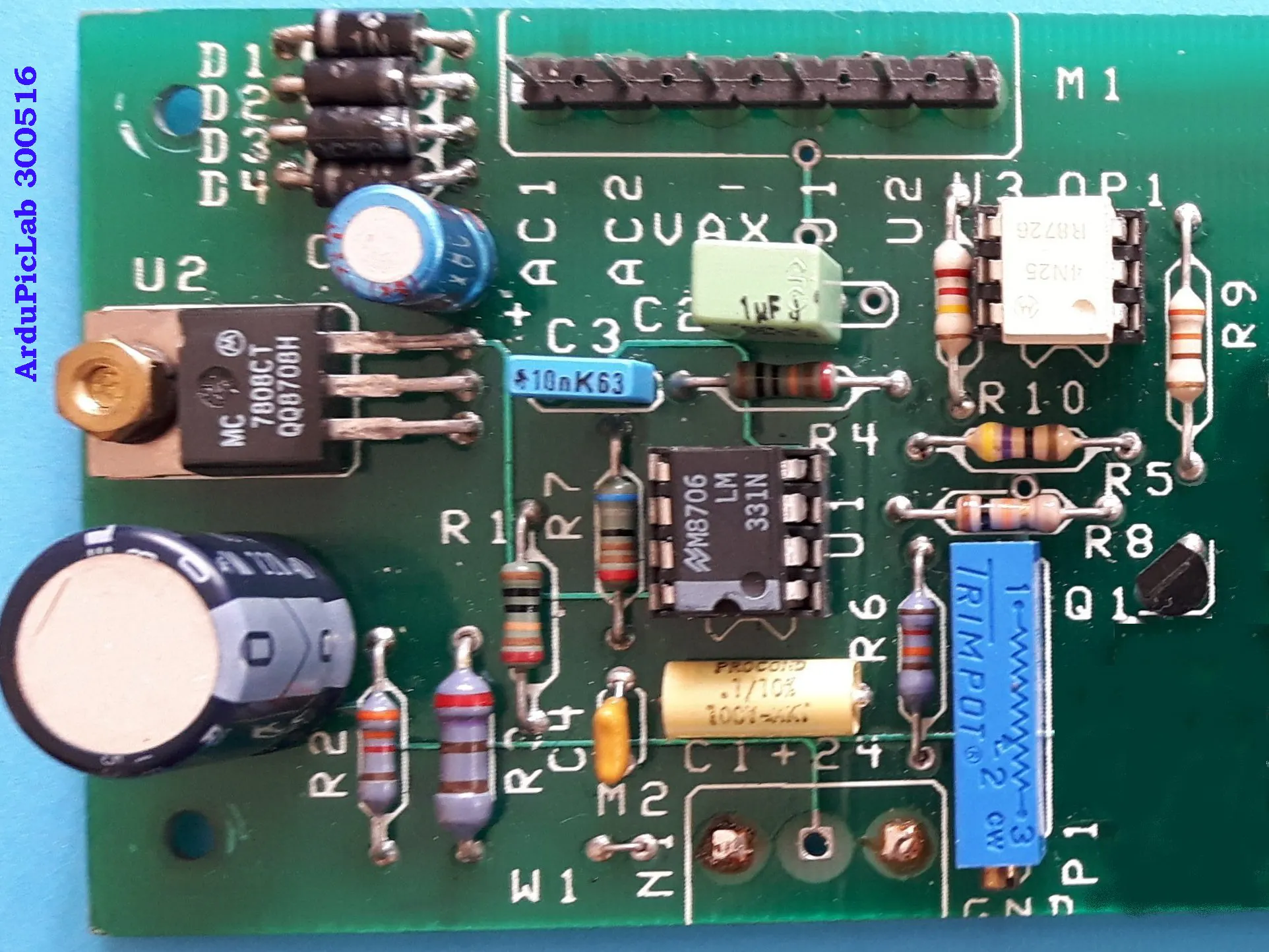## Things used in this project

### Hardware components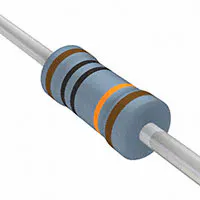Resistor 100k ohm ±1% metal film
×2Arduino UNO & Genuino UNO
×1
 Texas Instruments LM331
×1
 LM7808
×1
 resistor 3.3k ohm ±1% metal film
×1
 resistor 270 ohm ±1% metal film
×1
 resistor 47 ohm
×1
 resistor 12k ohm ±1% metal film
×1
 resistor 6.8k ohm ±1% metal film
×1Resistor 10k ohm
×1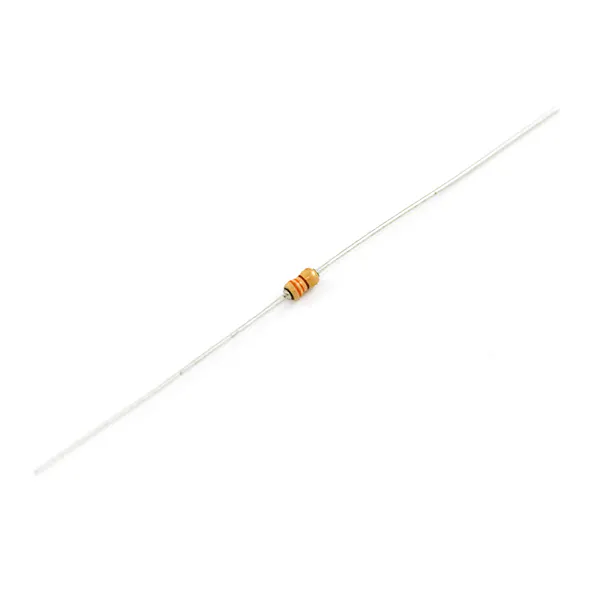Resistor 330 ohm
×1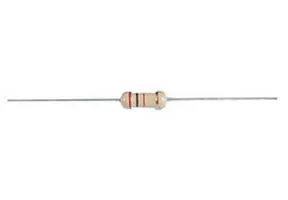Resistor 220 ohm
×1
 multi turn precision potentiometer 5k ohm
×1
 capacitor 100nF Mylar type
×1
 capacitor 10 nF Mylar type
×1
 capacitor 1 MF Mylar type
×1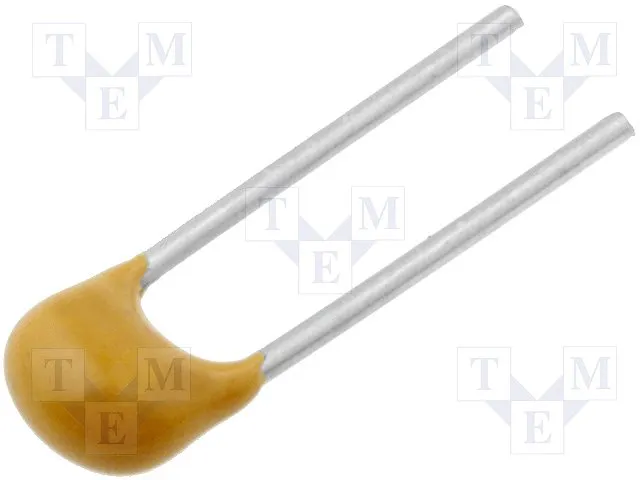Capacitor 100 nF
×1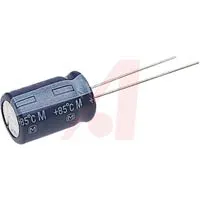Capacitor 4.7 µF
×1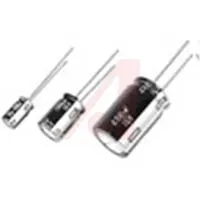Capacitor 220 µF
×1
 diode 1N4001
×4
 4N25 opto isolator
×1

### Software apps and online servicesArduino IDE

### Hand tools and fabrication machinesSoldering iron (generic)

## Schematics

### Schematic of the circuit

The circuit accepts an input voltage from about 20mV to 5V or a current of 4 to 20 mA (with the jumper W1 inserted). The two resistors in parallel R2 and R3 give a value of about 250 ohms, in order to have 1V to 5V for 4mA to 20mA current input.
Just three wires and a resistor are required to connect the circuit to the Arduino Uno. The output of the opto-coupler should be connected to the digital input D5 with a pull-up 2.2k resistor connected to the +5V of Arduino.
If an input range of 10V is required, a 15V power supply is necessary, so you have to change the 7808 regulator with a 7815. The transformer T1 also has to power supply the sensor, so it must have an adequate voltage and power. The trimmer P1 must be adjusted to obtain a conversion factor of about 1kHz/V.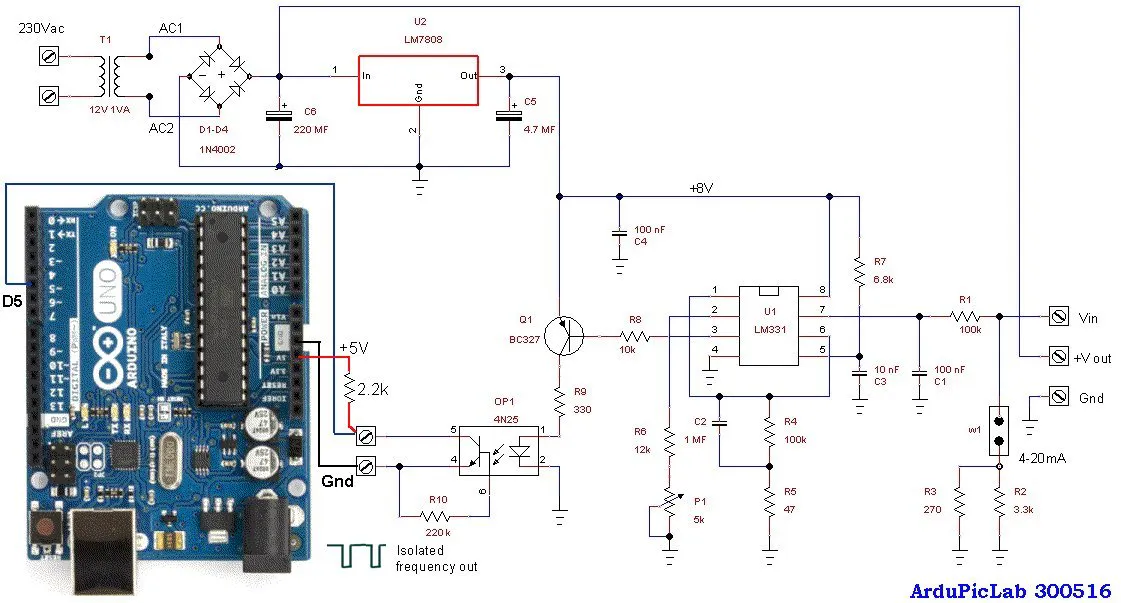## Code

### Arduino interface and program

Arduino
Arduino can measure the frequency in two ways:
• period measurement, using the pulsein() function;
• frequency measurement, with CPU Timer/Counters , using special libraries.
The first method uses twice the function pulsein() in order to measure the HIGH time and LOW time of the signal, with a microsecond resolution. The sum of the two measurements is the period of the signal. For a 5kHz signal, the period is 200 µs = HIGH time + LOW time = 125+75 µs. The time resolution is relatively low and the accuracy of measurement is also affected by the time of the program instructions.
The positive aspect about this method is the measurement speed which is slightly higher than the measured period. At a disadvantage, in addition to the less accuracy, it is most sensitive to the electrical grid noise (50 or 60Hz).
For these reasons, I prefer a frequency measurement with respect to the period. The measurement time is higher but you get a higher precision and precise sampling times. In addition, choosing a measurement period multiple of that of the electrical grid, it has excellent noise immunity.
I use the FreqCounter library by Martin Nawrath KHM LAB3:
This library uses Timer/Counter1 for counting pulses on rising edge of T1/PD5/digitalPin5 and Timer/Counter2 for the gate-time generation with 1 ms interrupts.
I chose a gate time equal to 1000 ms to mediate the count on a period of 50 or 60 mains cycles. In this case you get a resolution that is five times greater than that of Arduino Uno.
```#include <FreqCounter.h>

void setup() {
Serial.begin(9600);  // connect to the serial port
}

long int frq;
void loop() {

FreqCounter::f_comp= 0;   // Set compensation to 0
FreqCounter::start(1000);  // Start counting with gatetime of 1000ms